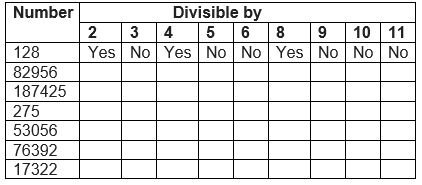# Important questions for Class 6 Maths Playing With Numbers

In this page we have Important questions for Class 6 Maths Playing With Numbers . Hope you like them and do not forget to like , social share and comment at the end of the page.
Question 1
Sort out even and odd numbers: 13, 28, 41, 99, 50, 255, 132, 664, 59, 29, 71, 165, 14

Question 2
X completes one round of a running track in 8 minutes and Y completes it in 6 minutes. How long will it take for both to arrive at their starting point together, if they start at the same time and maintain their speed?

Question 3
Find the HCF of 70, 105, 175.

Question 4
Determine the smallest three-digit number which is exactly divisible by 6, 8 and 12.

Question 5
Find the prime factorization of 4900

Question 6
Find the L.C.M of the following by prime factorization method:
(i) 42, 63, 162
(ii) 42, 78, 104, 112
(iii) 16, 28, 40, 77
(iv) 112, 168, 22824.

Question 7
Write seven consecutive composite numbers less than 100 so that there is no prime number between them.

Question 8
The length, breadth and height of a room are 825cm, 675cm and 450cm respectively. Find the longest tape which can measure the three dimensions of the room exactly.

Question 9
Using divisibility test, determine which of the following numbers are divisible by 2; by 3; by 4; by 5; by 6; by 8; by 9; by 10; by 11.Question 10
Find the HCF of the following numbers:
(a) 16, 48
(b) 10, 42
(c) 20, 60
(d) 18, 63
(e) 36, 84
(f) 32, 108
(g) 70, 115, 175
(h) 91, 112, 49
(i) 18, 54, 81
(j) 12, 45, 85

Question 11
Fill in the blanks
a. Every multiple of a given number is greater than or equal to that ________.
b. The number _____ is the smallest prime number and is even.
c. HCF of 10 and 15 is _____.
d. First three multiples of 11 are ________.
e. The HCF of two co- prime numbers is __________.
f. The LCM of two co-prime numbers is __________.
g. If two given numbers are divisible by a number, then their _______ and ________also divisible by that number.
h. A number is divisible by ______, if it is divisible by both 3 and 6.
i. HCF of 11 and 44 is _____.
j. The numbers which are not multiple of 2 are called ________ numbers.
k. The numbers which are a multiple of 2 are called ______ numbers.
l. First two multiples of 8 are ________.### Practice Question

Question 1 What is $\frac {1}{2} + \frac {3}{4}$ ?
A)$\frac {5}{4}$
B)$\frac {1}{4}$
C)$1$
D)$\frac {4}{5}$
Question 2 Pinhole camera produces an ?
A)An erect and small image
B)an Inverted and small image
C)An inverted and enlarged image
D)None of the above